Скачать презентацию Semiconductor Industry Increasing miniaturization Moore s Law is leading

2123ca162f9592a83f5992cda06e48c8.ppt

• Количество слайдов: 62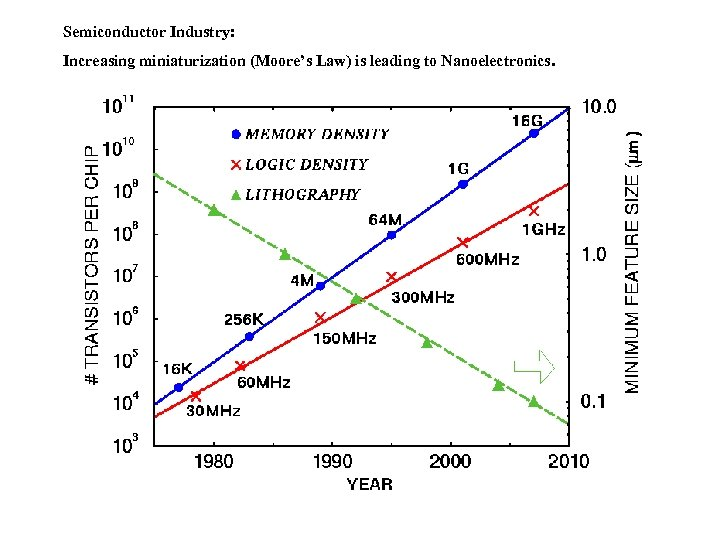Semiconductor Industry: Increasing miniaturization (Moore’s Law) is leading to Nanoelectronics.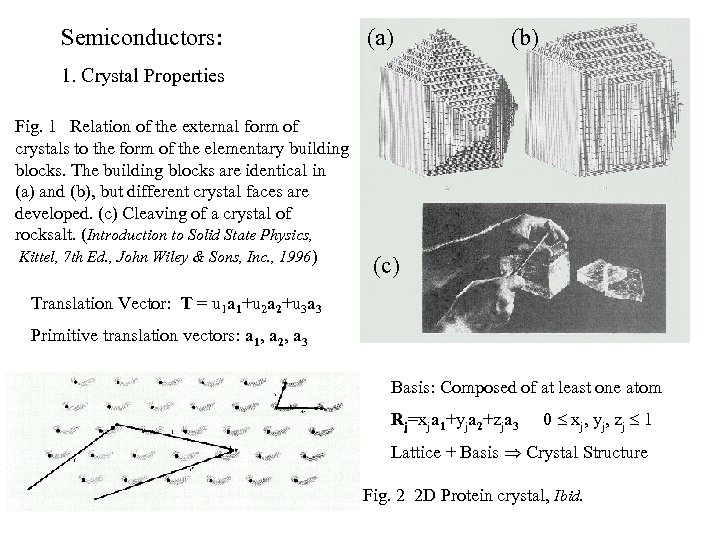Semiconductors: (a) (b) 1. Crystal Properties Fig. 1 Relation of the external form of crystals to the form of the elementary building blocks. The building blocks are identical in (a) and (b), but different crystal faces are developed. (c) Cleaving of a crystal of rocksalt. (Introduction to Solid State Physics, Kittel, 7 th Ed. , John Wiley & Sons, Inc. , 1996) (c) Translation Vector: T = u 1 a 1+u 2 a 2+u 3 a 3 Primitive translation vectors: a 1, a 2, a 3 Basis: Composed of at least one atom Rj=xja 1+yja 2+zja 3 0 xj, yj, zj 1 Lattice + Basis Crystal Structure Fig. 2 2 D Protein crystal, Ibid.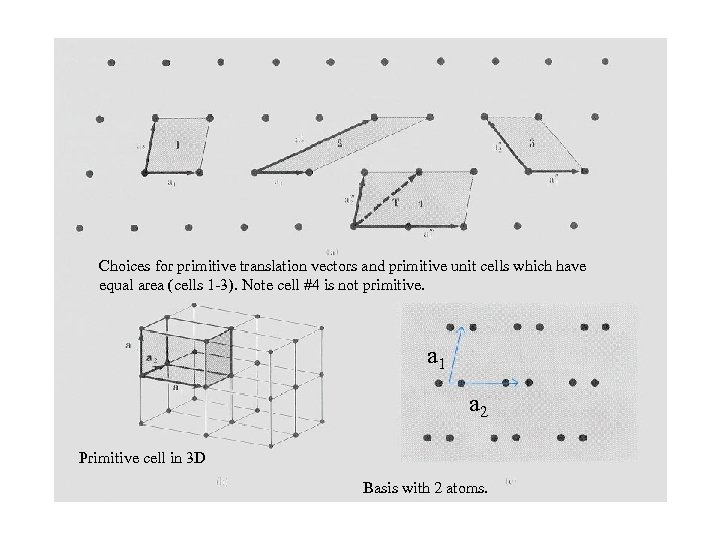Choices for primitive translation vectors and primitive unit cells which have equal area (cells 1 -3). Note cell #4 is not primitive. a 1 a 2 Primitive cell in 3 D Basis with 2 atoms.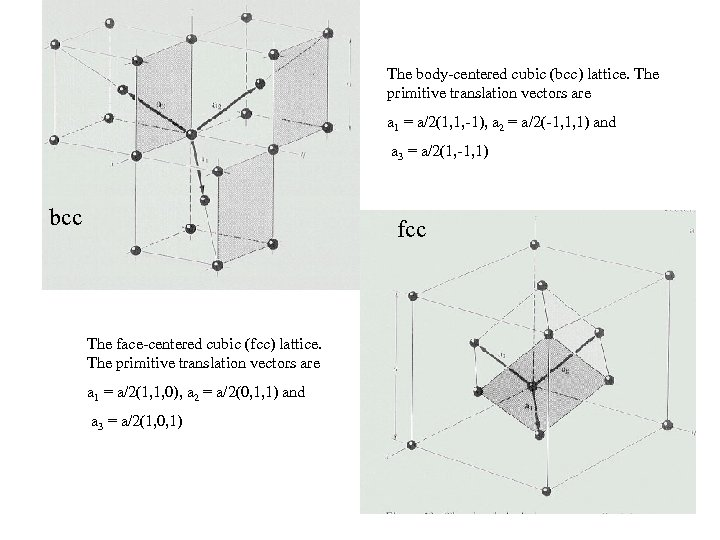The body-centered cubic (bcc) lattice. The primitive translation vectors are a 1 = a/2(1, 1, -1), a 2 = a/2(-1, 1, 1) and a 3 = a/2(1, -1, 1) bcc fcc The face-centered cubic (fcc) lattice. The primitive translation vectors are a 1 = a/2(1, 1, 0), a 2 = a/2(0, 1, 1) and a 3 = a/2(1, 0, 1)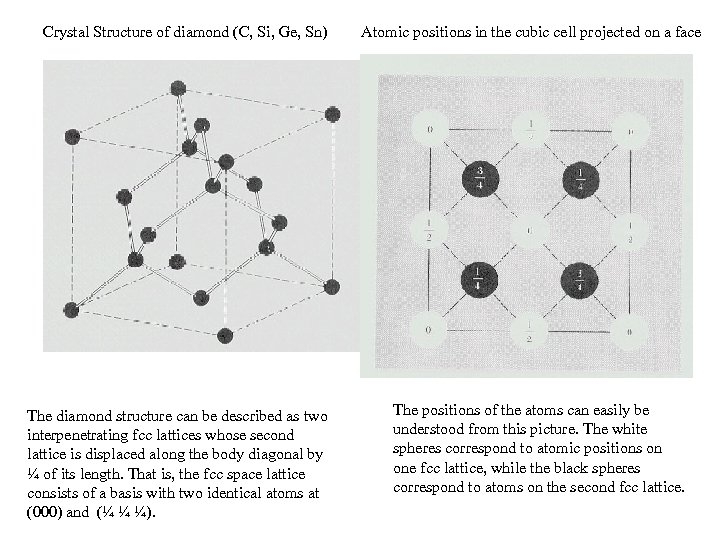Crystal Structure of diamond (C, Si, Ge, Sn) The diamond structure can be described as two interpenetrating fcc lattices whose second lattice is displaced along the body diagonal by ¼ of its length. That is, the fcc space lattice consists of a basis with two identical atoms at (000) and (¼ ¼ ¼). Atomic positions in the cubic cell projected on a face The positions of the atoms can easily be understood from this picture. The white spheres correspond to atomic positions on one fcc lattice, while the black spheres correspond to atoms on the second fcc lattice.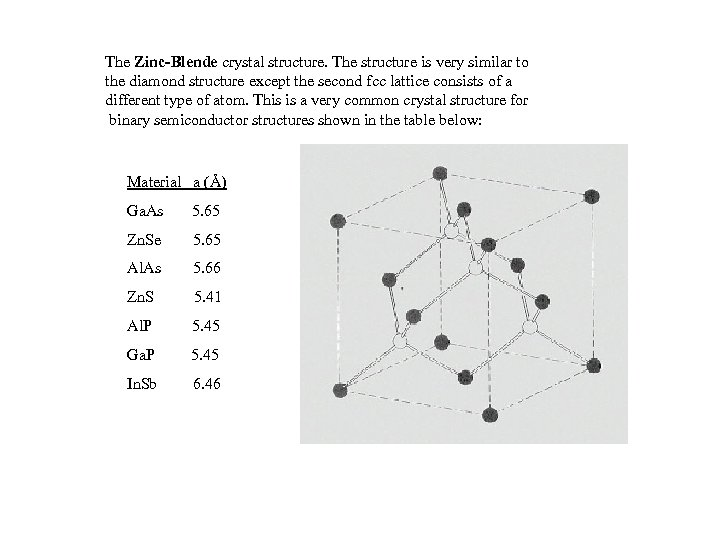The Zinc-Blende crystal structure. The structure is very similar to the diamond structure except the second fcc lattice consists of a different type of atom. This is a very common crystal structure for binary semiconductor structures shown in the table below: Material a (Å) Ga. As 5. 65 Zn. Se 5. 65 Al. As 5. 66 Zn. S 5. 41 Al. P 5. 45 Ga. P 5. 45 In. Sb 6. 46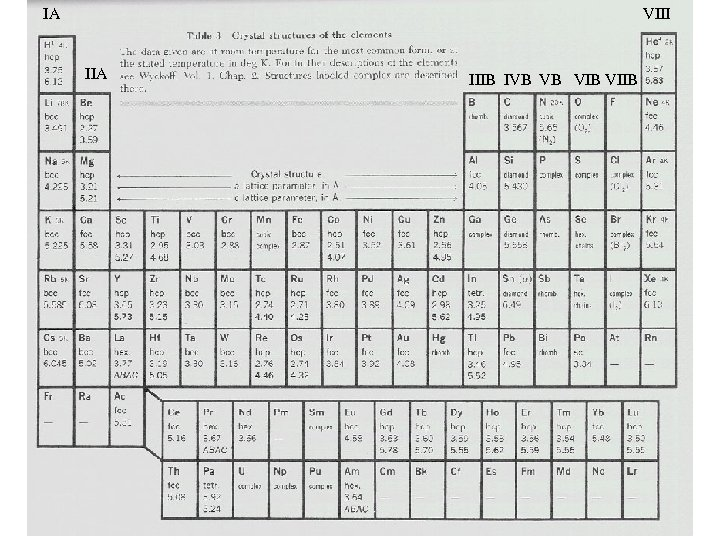IA VIII IIA IIIB IVB VB VIIB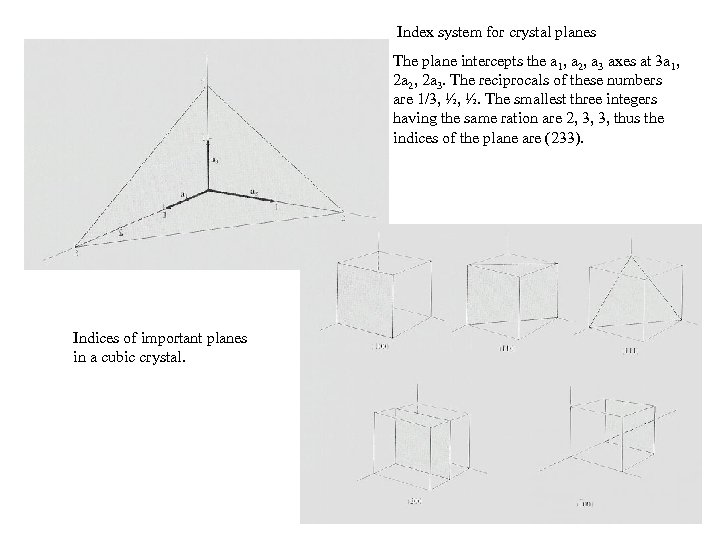Index system for crystal planes The plane intercepts the a 1, a 2, a 3 axes at 3 a 1, 2 a 2, 2 a 3. The reciprocals of these numbers are 1/3, ½, ½. The smallest three integers having the same ration are 2, 3, 3, thus the indices of the plane are (233). Indices of important planes in a cubic crystal.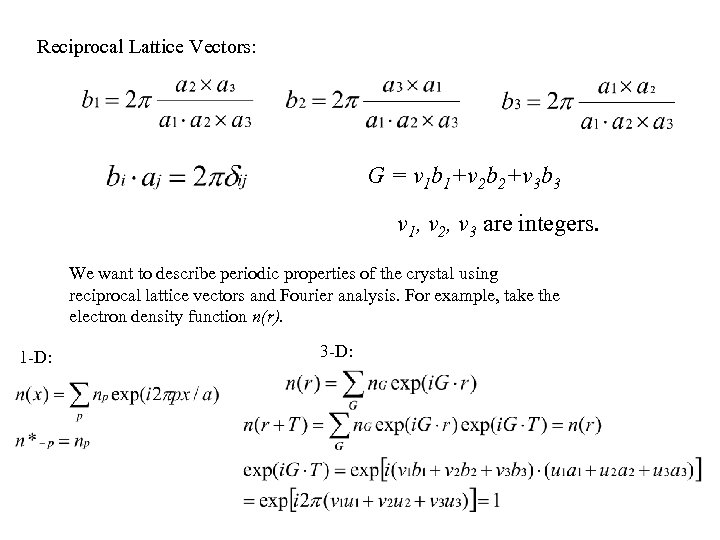Reciprocal Lattice Vectors: G = v 1 b 1+v 2 b 2+v 3 b 3 v 1, v 2, v 3 are integers. We want to describe periodic properties of the crystal using reciprocal lattice vectors and Fourier analysis. For example, take the electron density function n(r). 1 -D: 3 -D: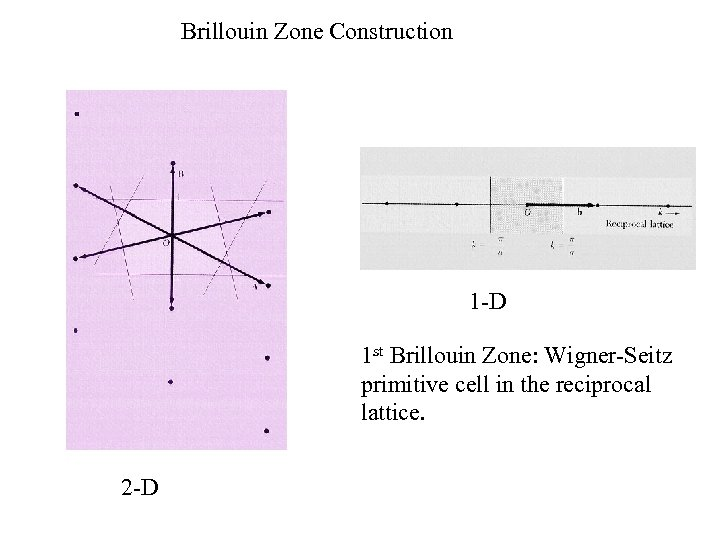Brillouin Zone Construction 1 -D 1 st Brillouin Zone: Wigner-Seitz primitive cell in the reciprocal lattice. 2 -D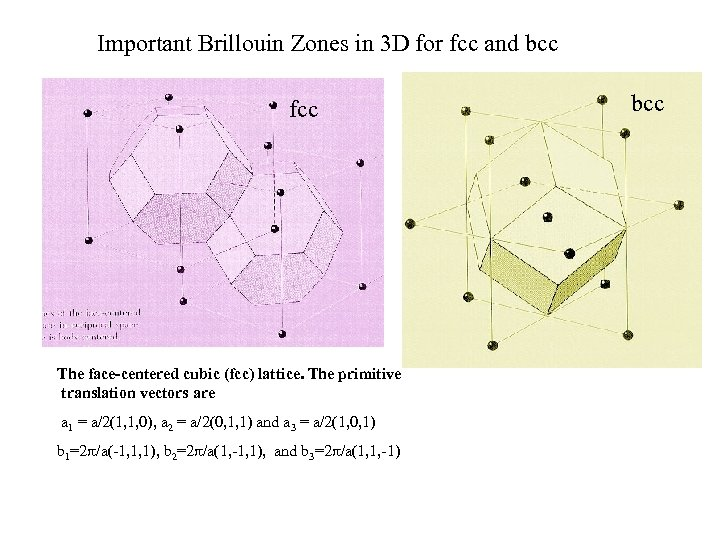Important Brillouin Zones in 3 D for fcc and bcc fcc The face-centered cubic (fcc) lattice. The primitive translation vectors are a 1 = a/2(1, 1, 0), a 2 = a/2(0, 1, 1) and a 3 = a/2(1, 0, 1) b 1=2 /a(-1, 1, 1), b 2=2 /a(1, -1, 1), and b 3=2 /a(1, 1, -1) bcc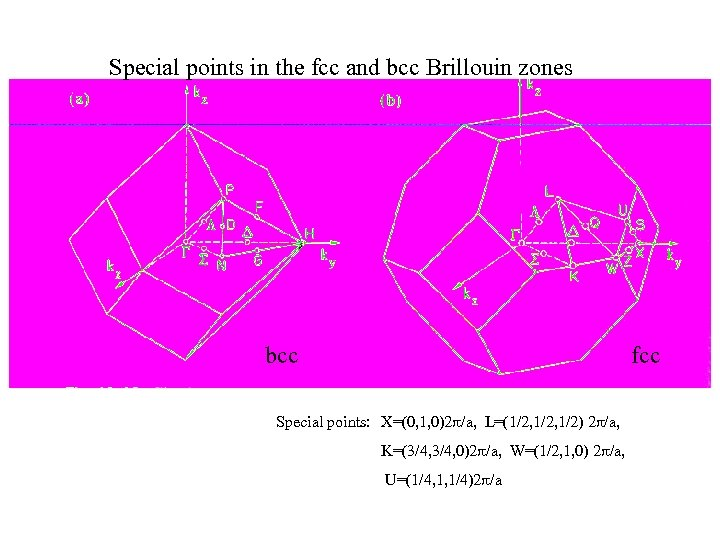Special points in the fcc and bcc Brillouin zones bcc fcc Special points: X=(0, 1, 0)2 /a, L=(1/2, 1/2) 2 /a, K=(3/4, 0)2 /a, W=(1/2, 1, 0) 2 /a, U=(1/4, 1, 1/4)2 /a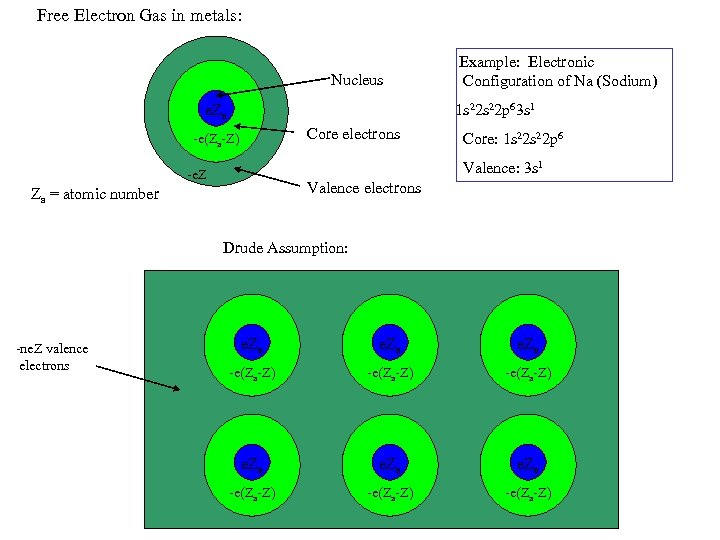Free Electron Gas in metals: Nucleus Example: Electronic Configuration of Na (Sodium) 1 s 22 p 63 s 1 e. Za Core electrons -e(Za-Z) Core: 1 s 22 p 6 Valence: 3 s 1 -e. Z Valence electrons Za = atomic number Drude Assumption: -ne. Z valence electrons e. Za -e(Za-Z) -e(Za-Z)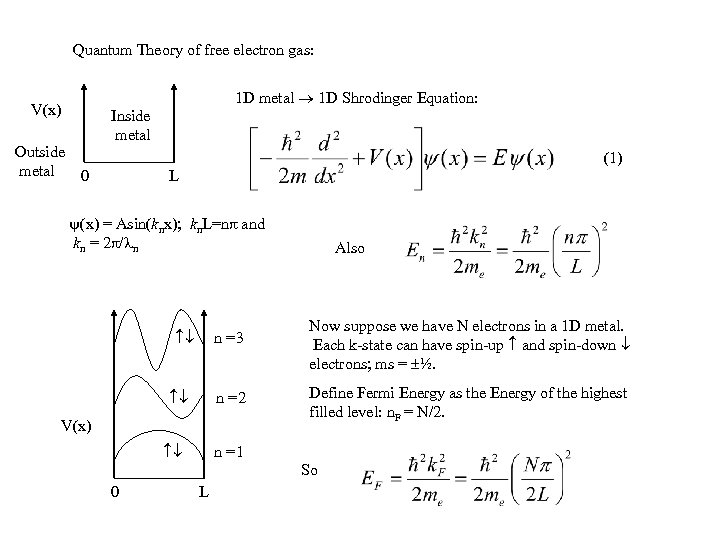Quantum Theory of free electron gas: 1 D metal 1 D Shrodinger Equation: V(x) Outside metal 0 Inside metal (1) L (x) = Asin(knx); kn. L=n and kn = 2 / n Also n =3 Now suppose we have N electrons in a 1 D metal. Each k-state can have spin-up and spin-down electrons; ms = ½. n =2 Define Fermi Energy as the Energy of the highest filled level: n. F = N/2. V(x) n =1 So 0 L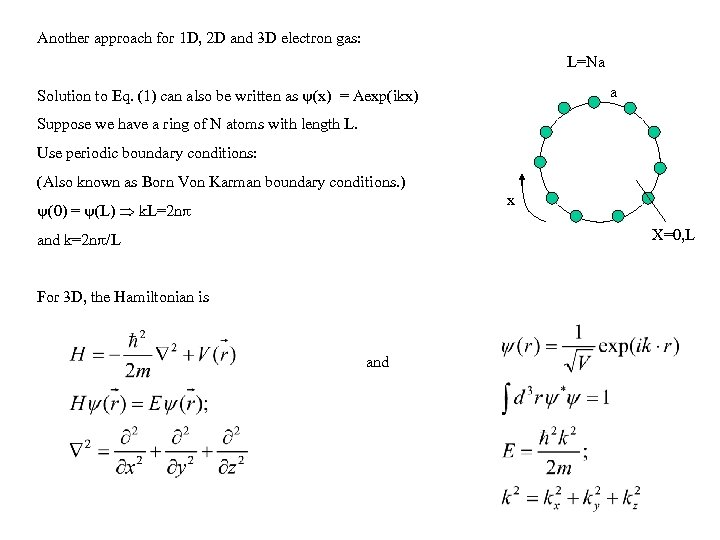Another approach for 1 D, 2 D and 3 D electron gas: L=Na a Solution to Eq. (1) can also be written as (x) = Aexp(ikx) Suppose we have a ring of N atoms with length L. Use periodic boundary conditions: (Also known as Born Von Karman boundary conditions. ) x (0) = (L) k. L=2 n X=0, L and k=2 n /L For 3 D, the Hamiltonian is and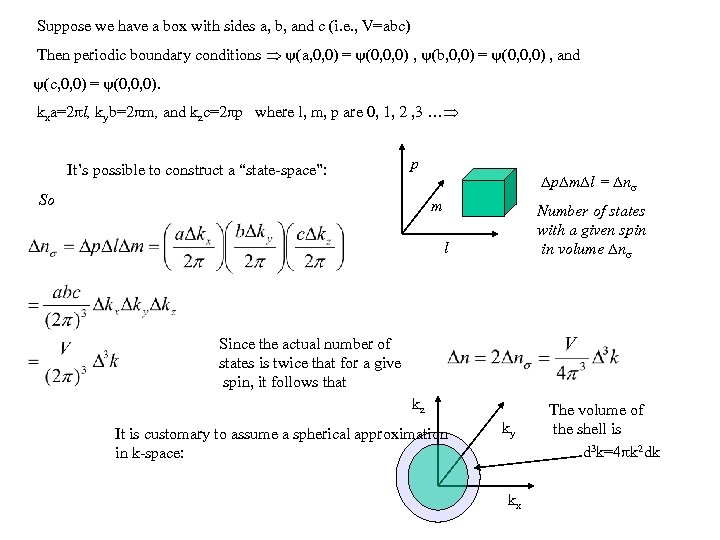Suppose we have a box with sides a, b, and c (i. e. , V=abc) Then periodic boundary conditions (a, 0, 0) = (0, 0, 0) , (b, 0, 0) = (0, 0, 0) , and (c, 0, 0) = (0, 0, 0). kxa=2 l, kyb=2 m, and kzc=2 p where l, m, p are 0, 1, 2 , 3 … It’s possible to construct a “state-space”: p So p m l = n m Number of states with a given spin in volume n l Since the actual number of states is twice that for a give spin, it follows that kz It is customary to assume a spherical approximation in k-space: ky kx The volume of the shell is d 3 k=4 k 2 dk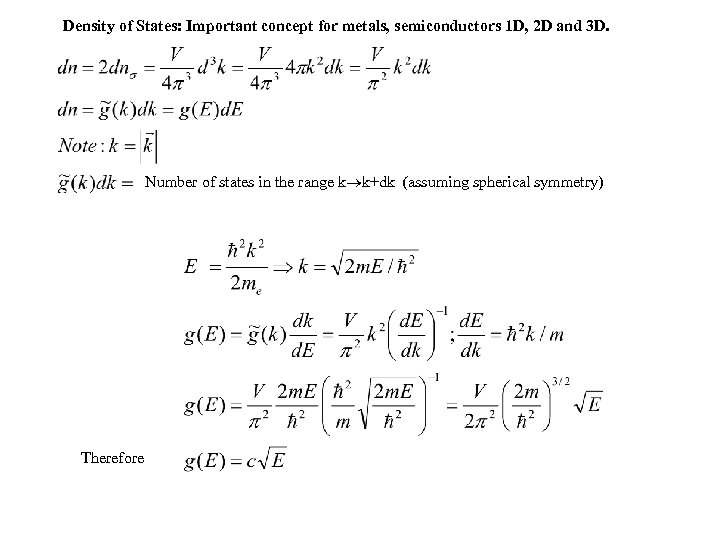Density of States: Important concept for metals, semiconductors 1 D, 2 D and 3 D. Number of states in the range k k+dk (assuming spherical symmetry) Therefore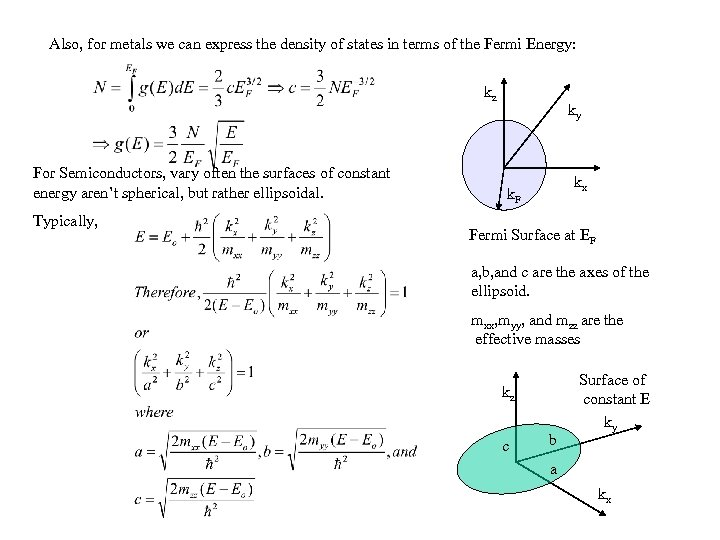Also, for metals we can express the density of states in terms of the Fermi Energy: kz For Semiconductors, vary often the surfaces of constant energy aren’t spherical, but rather ellipsoidal. Typically, ky kx k. F Fermi Surface at EF a, b, and c are the axes of the ellipsoid. mxx, myy, and mzz are the effective masses kz c b Surface of constant E ky a kx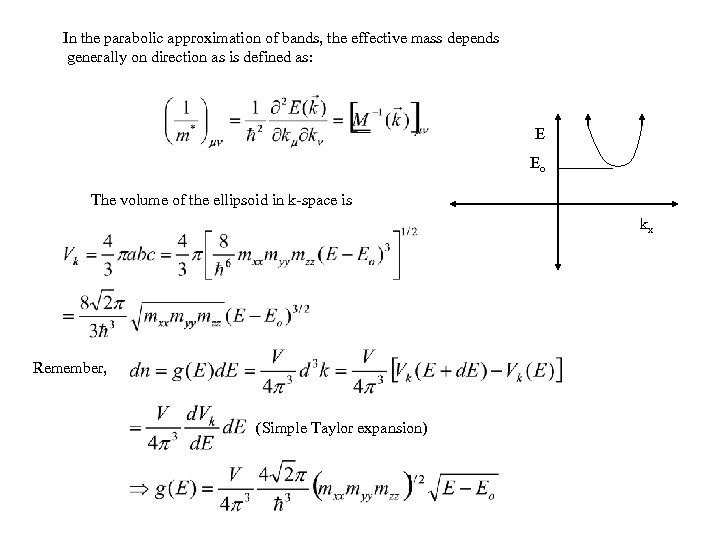In the parabolic approximation of bands, the effective mass depends generally on direction as is defined as: E Eo The volume of the ellipsoid in k-space is kx Remember, (Simple Taylor expansion)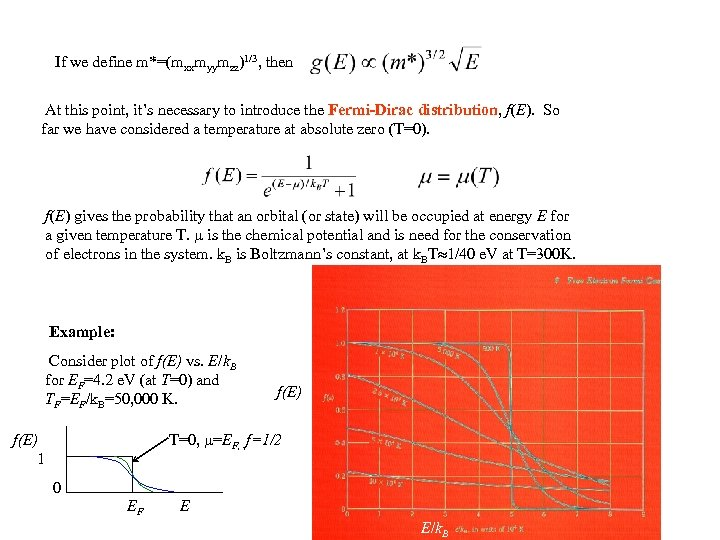If we define m*=(mxxmyymzz)1/3, then At this point, it’s necessary to introduce the Fermi-Dirac distribution, f(E). So far we have considered a temperature at absolute zero (T=0). f(E) gives the probability that an orbital (or state) will be occupied at energy E for a given temperature T. is the chemical potential and is need for the conservation of electrons in the system. k. B is Boltzmann’s constant, at k. BT 1/40 e. V at T=300 K. Example: Consider plot of f(E) vs. E/k. B for EF=4. 2 e. V (at T=0) and TF=EF/k. B=50, 000 K. f(E) T=0, =EF, , f=1/2 f(E) 1 0 EF E E/k. B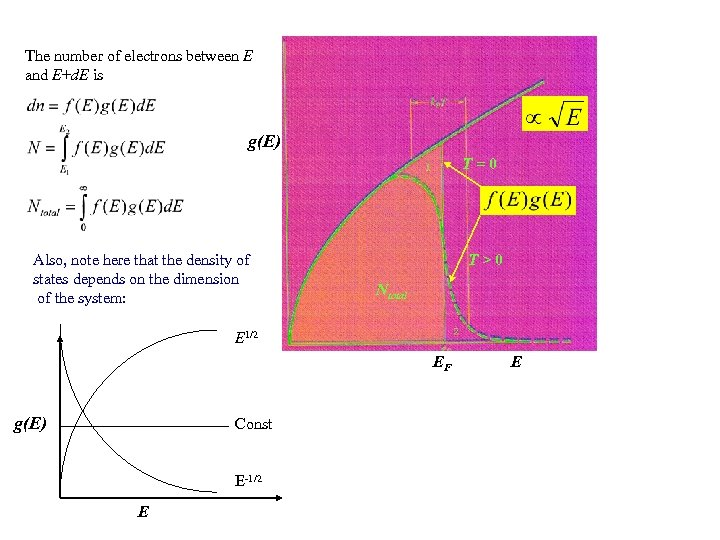The number of electrons between E and E+d. E is g(E) T=0 Also, note here that the density of states depends on the dimension of the system: T>0 Ntotal E 1/2 EF g(E) Const E-1/2 E E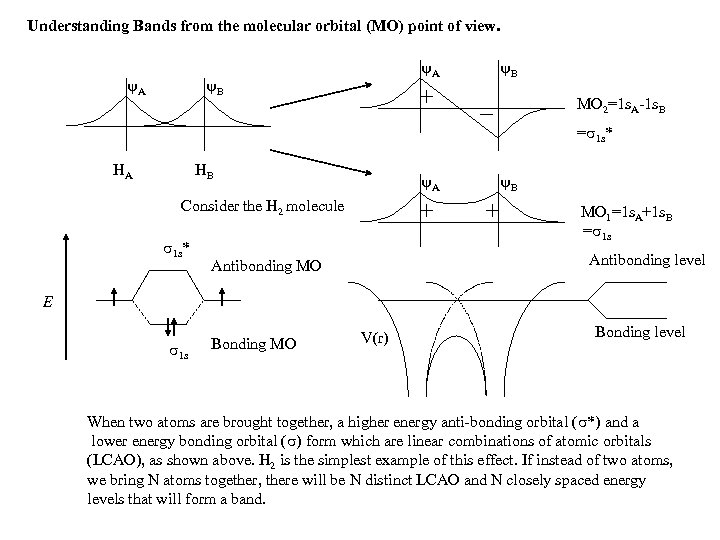Understanding Bands from the molecular orbital (MO) point of view. A A B B MO 2=1 s. A-1 s. B = 1 s* HA HB A Consider the H 2 molecule 1 s* B MO 1=1 s. A+1 s. B = 1 s Antibonding level Antibonding MO E 1 s Bonding MO V(r) Bonding level When two atoms are brought together, a higher energy anti-bonding orbital ( *) and a lower energy bonding orbital ( ) form which are linear combinations of atomic orbitals (LCAO), as shown above. H 2 is the simplest example of this effect. If instead of two atoms, we bring N atoms together, there will be N distinct LCAO and N closely spaced energy levels that will form a band.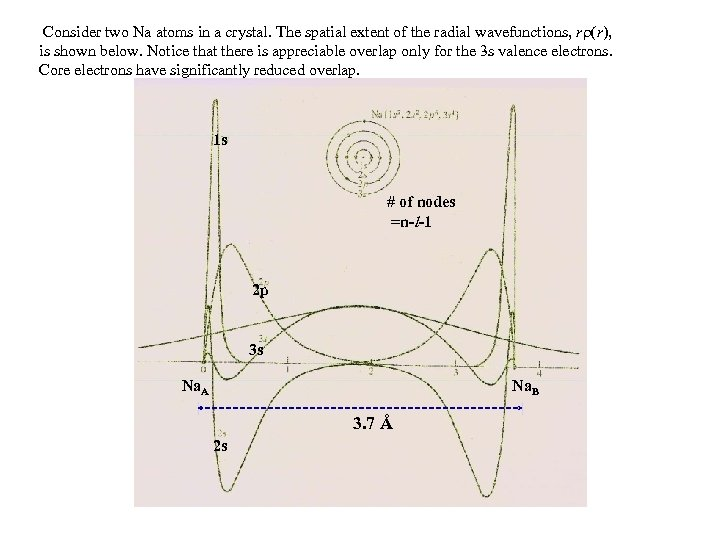Consider two Na atoms in a crystal. The spatial extent of the radial wavefunctions, r (r), is shown below. Notice that there is appreciable overlap only for the 3 s valence electrons. Core electrons have significantly reduced overlap. 1 s # of nodes =n-l-1 2 p 3 s Na. A Na. B 3. 7 Å 2 s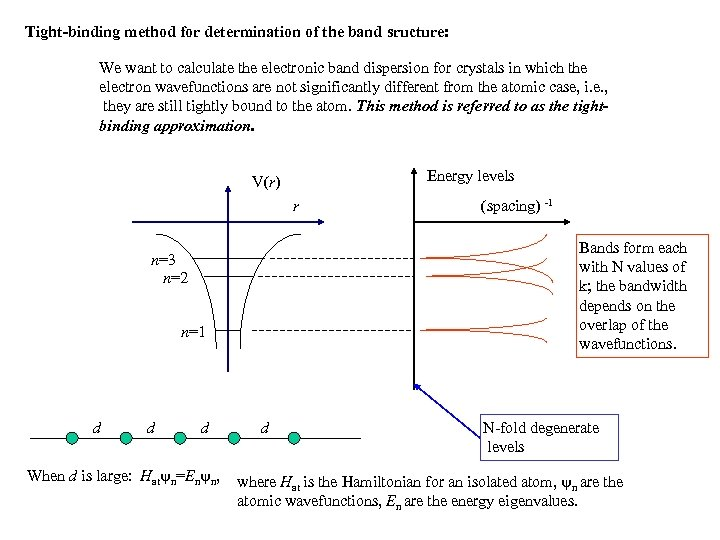Tight-binding method for determination of the band sructure: We want to calculate the electronic band dispersion for crystals in which the electron wavefunctions are not significantly different from the atomic case, i. e. , they are still tightly bound to the atom. This method is referred to as the tightbinding approximation. Energy levels V(r) r Bands form each with N values of k; the bandwidth depends on the overlap of the wavefunctions. n=3 n=2 n=1 d d d When d is large: Hat n=En n, (spacing) -1 d N-fold degenerate levels where Hat is the Hamiltonian for an isolated atom, n are the atomic wavefunctions, En are the energy eigenvalues.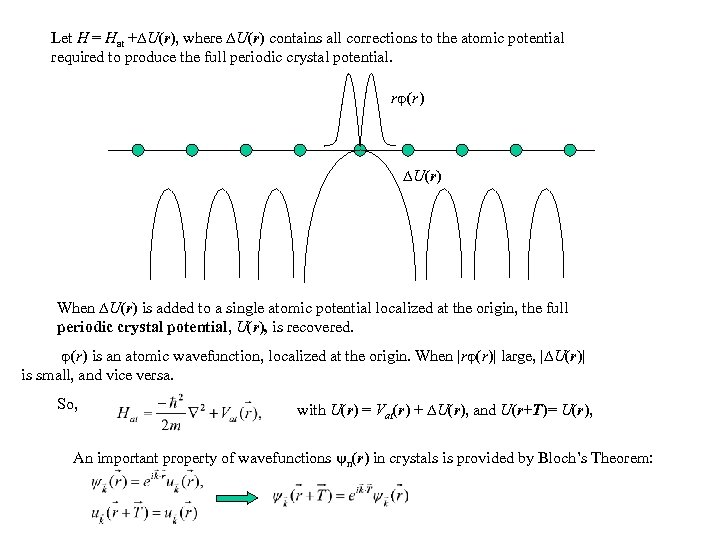Let H = Hat + U(r), where U(r) contains all corrections to the atomic potential required to produce the full periodic crystal potential. r (r ) U (r ) When U(r) is added to a single atomic potential localized at the origin, the full periodic crystal potential, U(r), is recovered. (r) is an atomic wavefunction, localized at the origin. When |r (r)| large, | U(r)| is small, and vice versa. So, with U(r) = Vat(r) + U(r), and U(r+T)= U(r), An important property of wavefunctions n(r) in crystals is provided by Bloch’s Theorem: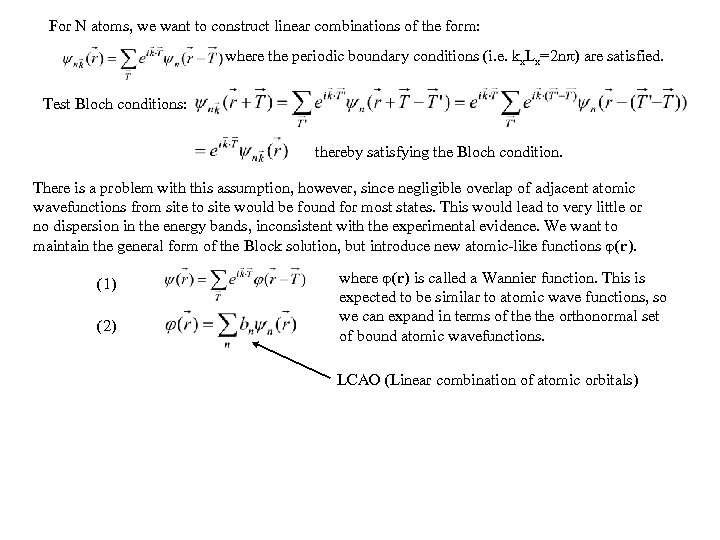For N atoms, we want to construct linear combinations of the form: where the periodic boundary conditions (i. e. kx. Lx=2 n ) are satisfied. Test Bloch conditions: thereby satisfying the Bloch condition. There is a problem with this assumption, however, since negligible overlap of adjacent atomic wavefunctions from site to site would be found for most states. This would lead to very little or no dispersion in the energy bands, inconsistent with the experimental evidence. We want to maintain the general form of the Block solution, but introduce new atomic-like functions (r). (1) (2) where (r) is called a Wannier function. This is expected to be similar to atomic wave functions, so we can expand in terms of the orthonormal set of bound atomic wavefunctions. LCAO (Linear combination of atomic orbitals)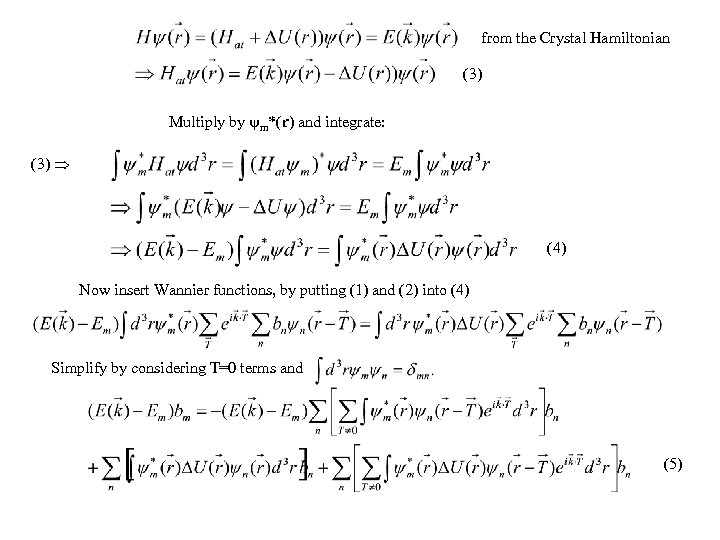from the Crystal Hamiltonian (3) Multiply by m*(r) and integrate: (3) (4) Now insert Wannier functions, by putting (1) and (2) into (4) Simplify by considering T=0 terms and (5)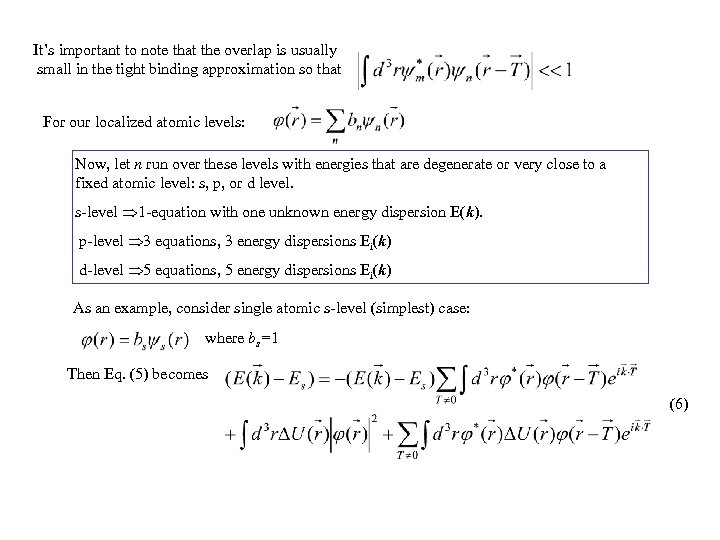It’s important to note that the overlap is usually small in the tight binding approximation so that For our localized atomic levels: Now, let n run over these levels with energies that are degenerate or very close to a fixed atomic level: s, p, or d level. s-level 1 -equation with one unknown energy dispersion E(k). p-level 3 equations, 3 energy dispersions Ei(k) d-level 5 equations, 5 energy dispersions Ei(k) As an example, consider single atomic s-level (simplest) case: where bs=1 Then Eq. (5) becomes (6)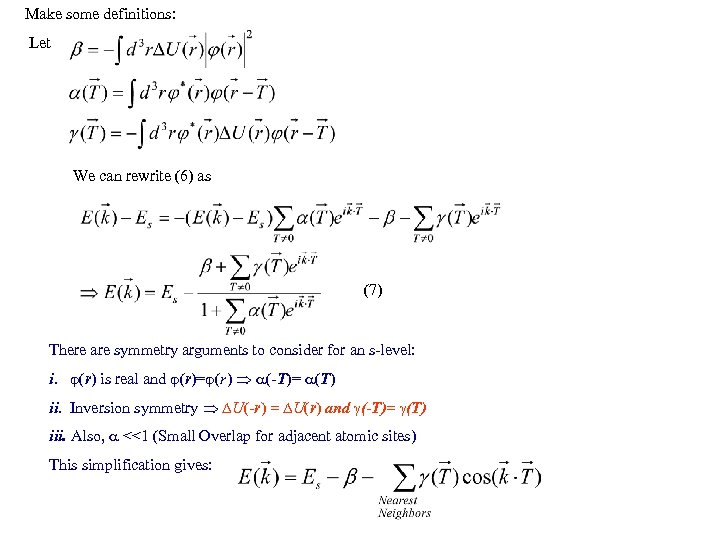Make some definitions: Let We can rewrite (6) as (7) There are symmetry arguments to consider for an s-level: i. (r) is real and (r)= (r) (-T)= (T) ii. Inversion symmetry U(-r) = U(r) and (-T)= (T) iii. Also, <<1 (Small Overlap for adjacent atomic sites) This simplification gives: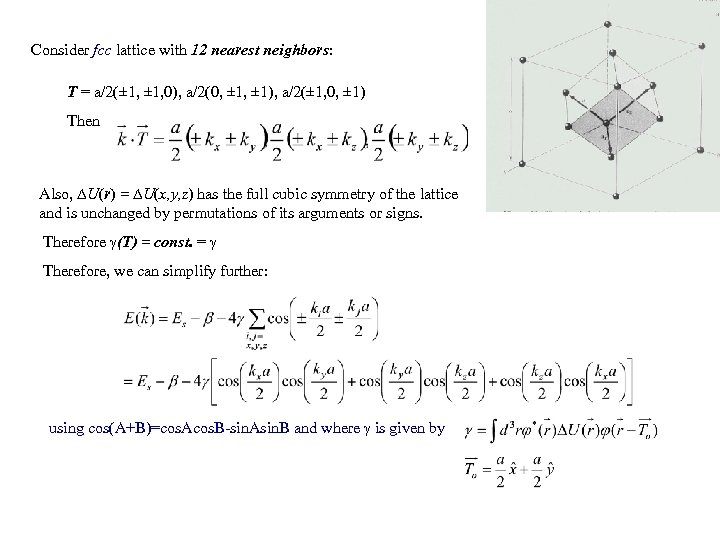Consider fcc lattice with 12 nearest neighbors: T = a/2(± 1, 0), a/2(0, ± 1), a/2(± 1, 0, ± 1) Then Also, U(r) = U(x, y, z) has the full cubic symmetry of the lattice and is unchanged by permutations of its arguments or signs. Therefore (T) = const. = Therefore, we can simplify further: using cos(A+B)=cos. Acos. B-sin. Asin. B and where is given by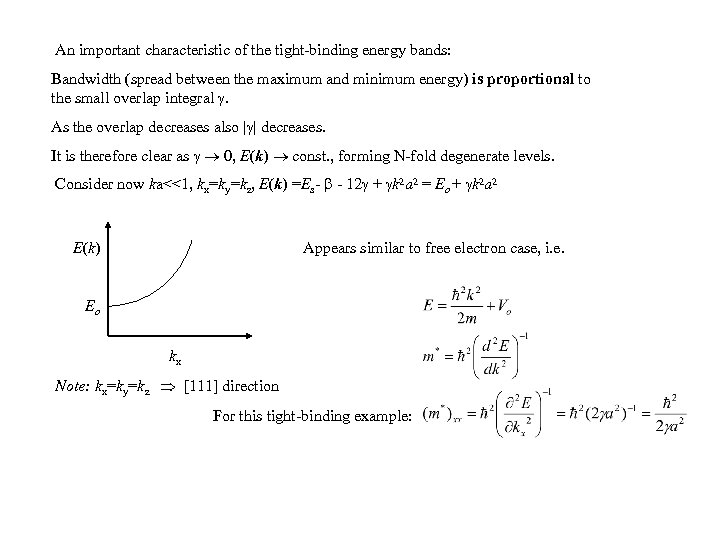An important characteristic of the tight-binding energy bands: Bandwidth (spread between the maximum and minimum energy) is proportional to the small overlap integral . As the overlap decreases also | | decreases. It is therefore clear as 0, E(k) const. , forming N-fold degenerate levels. Consider now ka<<1, kx=ky=kz, E(k) =Es- - 12 + k 2 a 2 = Eo + k 2 a 2 E (k ) Appears similar to free electron case, i. e. Eo kx Note: kx=ky=kz  direction For this tight-binding example: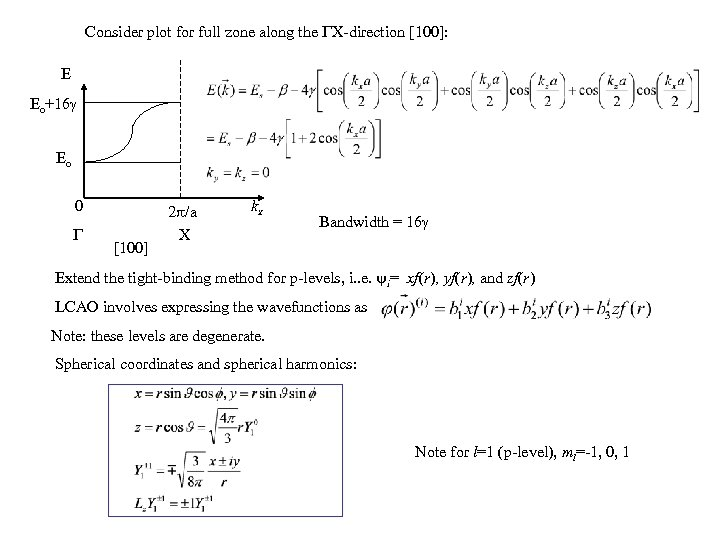Consider plot for full zone along the X-direction : E Eo+16 Eo 0  2 /a X kx Bandwidth = 16 Extend the tight-binding method for p-levels, i. . e. i= xf(r), yf(r), and zf(r) LCAO involves expressing the wavefunctions as Note: these levels are degenerate. Spherical coordinates and spherical harmonics: Note for l=1 (p-level), ml=-1, 0, 1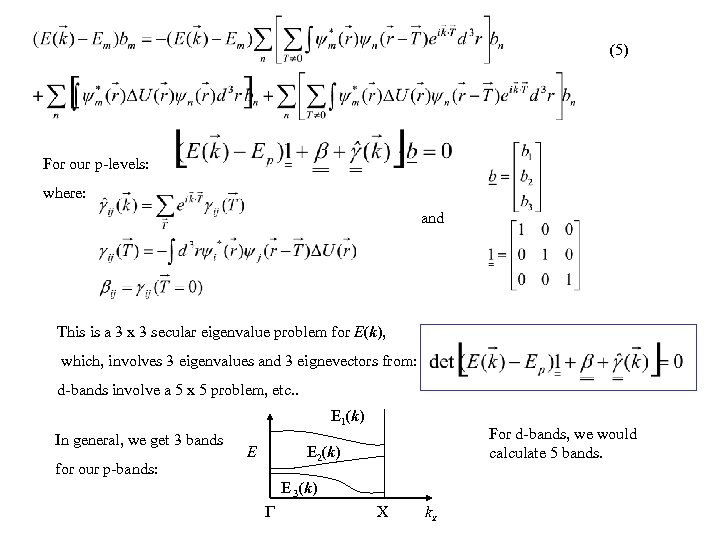(5) For our p-levels: where: and This is a 3 x 3 secular eigenvalue problem for E(k), which, involves 3 eigenvalues and 3 eignevectors from: d-bands involve a 5 x 5 problem, etc. . E 1(k) In general, we get 3 bands for our p-bands: E For d-bands, we would calculate 5 bands. E 2(k) E 3(k) X kx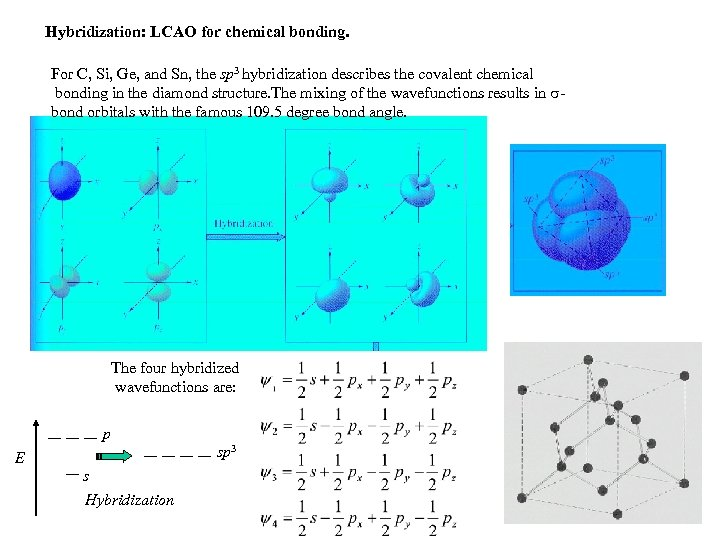Hybridization: LCAO for chemical bonding. For C, Si, Ge, and Sn, the sp 3 hybridization describes the covalent chemical bonding in the diamond structure. The mixing of the wavefunctions results in bond orbitals with the famous 109. 5 degree bond angle. The four hybridized wavefunctions are: p sp 3 E s Hybridization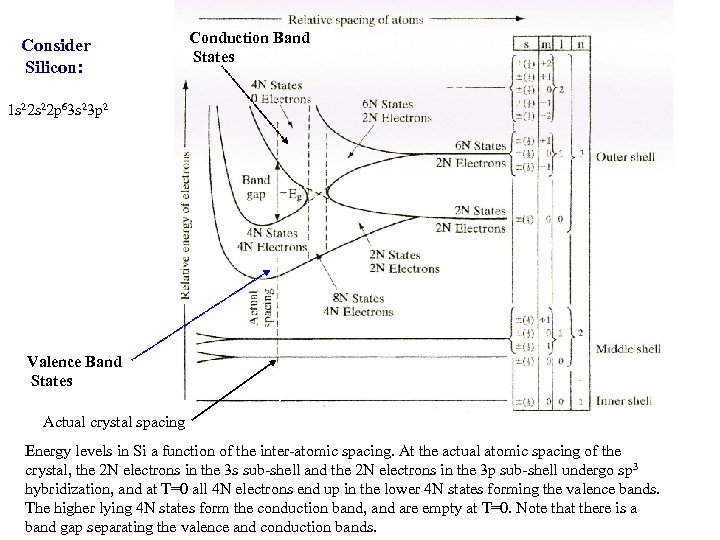Consider Silicon: Conduction Band States 1 s 22 p 63 s 23 p 2 Valence Band States Actual crystal spacing Energy levels in Si a function of the inter-atomic spacing. At the actual atomic spacing of the crystal, the 2 N electrons in the 3 s sub-shell and the 2 N electrons in the 3 p sub-shell undergo sp 3 hybridization, and at T=0 all 4 N electrons end up in the lower 4 N states forming the valence bands. The higher lying 4 N states form the conduction band, and are empty at T=0. Note that there is a band gap separating the valence and conduction bands.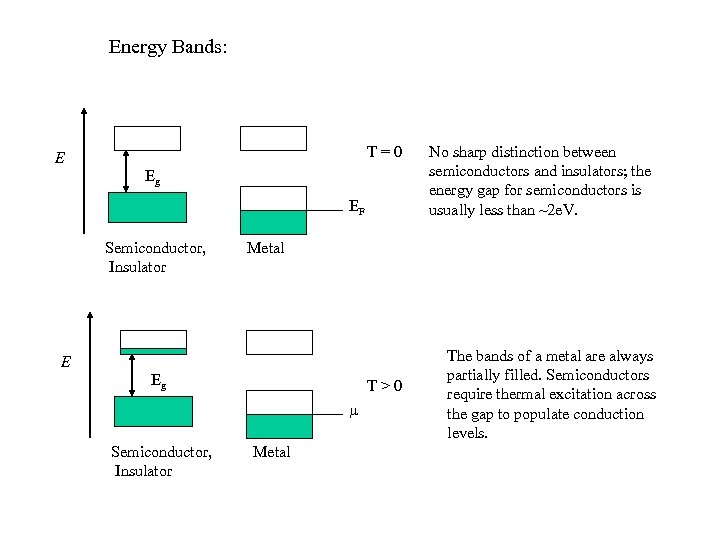Energy Bands: T=0 E Eg EF Semiconductor, Insulator Metal E Eg T>0 Semiconductor, Insulator No sharp distinction between semiconductors and insulators; the energy gap for semiconductors is usually less than ~2 e. V. Metal The bands of a metal are always partially filled. Semiconductors require thermal excitation across the gap to populate conduction levels.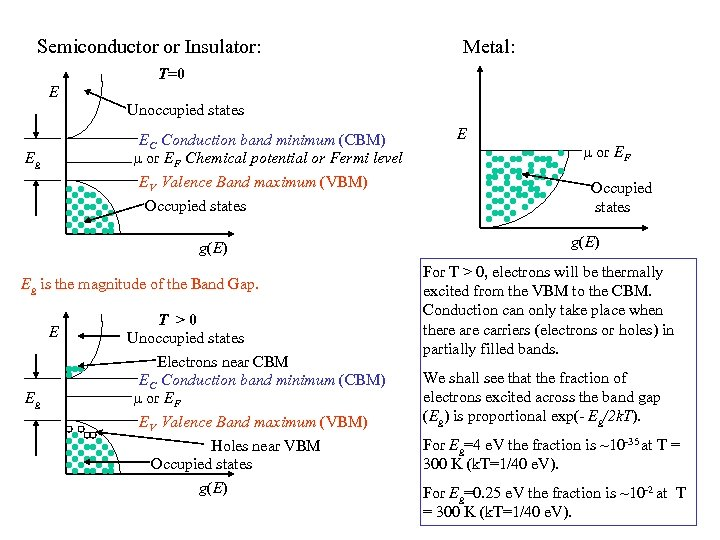Semiconductor or Insulator: Metal: T=0 E Unoccupied states EC Conduction band minimum (CBM) or EF Chemical potential or Fermi level EV Valence Band maximum (VBM) Occupied states Eg g(E) Eg is the magnitude of the Band Gap. E Eg T >0 Unoccupied states Electrons near CBM EC Conduction band minimum (CBM) or EF EV Valence Band maximum (VBM) Holes near VBM Occupied states g(E) E or EF Occupied states g(E) For T > 0, electrons will be thermally excited from the VBM to the CBM. Conduction can only take place when there are carriers (electrons or holes) in partially filled bands. We shall see that the fraction of electrons excited across the band gap (Eg) is proportional exp(- Eg/2 k. T). For Eg=4 e. V the fraction is ~10 -35 at T = 300 K (k. T=1/40 e. V). For Eg=0. 25 e. V the fraction is ~10 -2 at T = 300 K (k. T=1/40 e. V).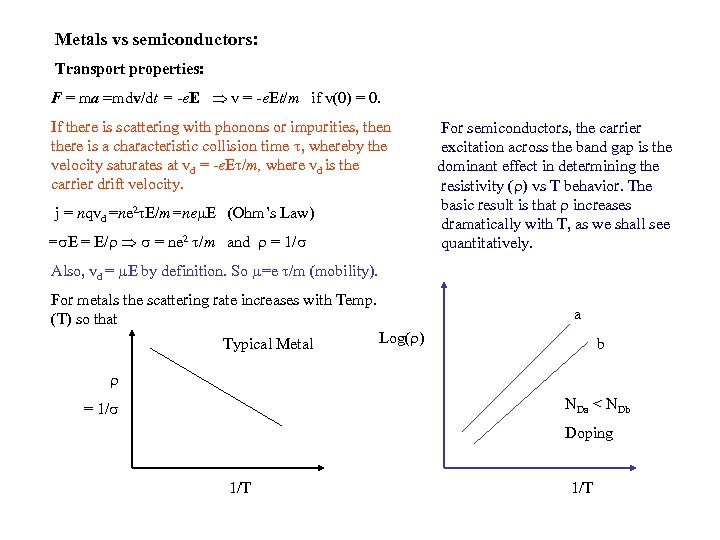Metals vs semiconductors: Transport properties: F = ma =mdv/dt = -e. E v = -e. Et/m if v(0) = 0. If there is scattering with phonons or impurities, then there is a characteristic collision time , whereby the velocity saturates at vd = -e. E /m, where vd is the carrier drift velocity. j = nqvd =ne 2 E/m=ne E (Ohm’s Law) = E/ = ne 2 /m and = 1/ For semiconductors, the carrier excitation across the band gap is the dominant effect in determining the resistivity ( ) vs T behavior. The basic result is that increases dramatically with T, as we shall see quantitatively. Also, vd = E by definition. So =e /m (mobility). For metals the scattering rate increases with Temp. (T) so that Typical Metal a Log( ) b NDa < NDb = 1/ Doping 1/T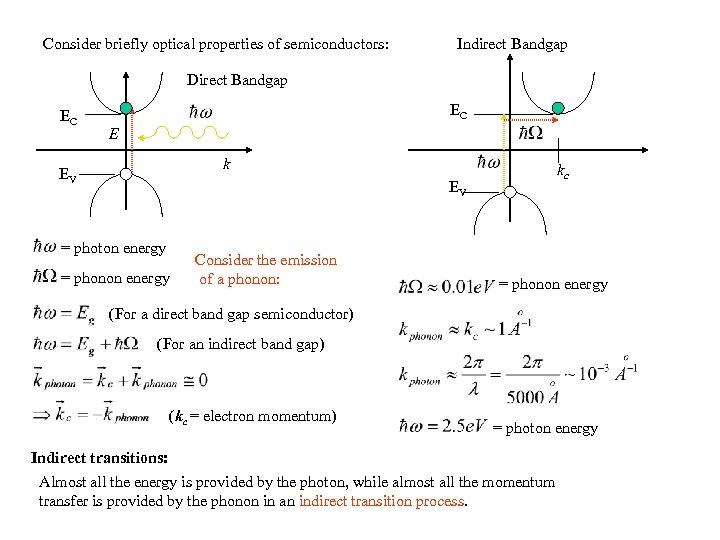Consider briefly optical properties of semiconductors: Indirect Bandgap Direct Bandgap EC EC E k EV kc EV = photon energy = phonon energy Consider the emission of a phonon: = phonon energy (For a direct band gap semiconductor) (For an indirect band gap) (kc = electron momentum) = photon energy Indirect transitions: Almost all the energy is provided by the photon, while almost all the momentum transfer is provided by the phonon in an indirect transition process.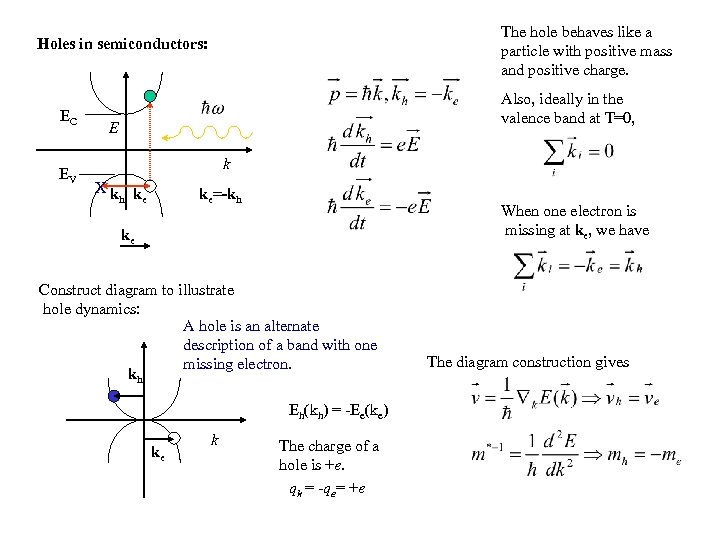The hole behaves like a particle with positive mass and positive charge. Holes in semiconductors: EC EV Also, ideally in the valence band at T=0, E k Xk k h e ke=-kh When one electron is missing at ke, we have ke Construct diagram to illustrate hole dynamics: A hole is an alternate description of a band with one missing electron. kh Eh(kh) = -Ee(ke) ke k The charge of a hole is +e. qh = -qe= +e The diagram construction gives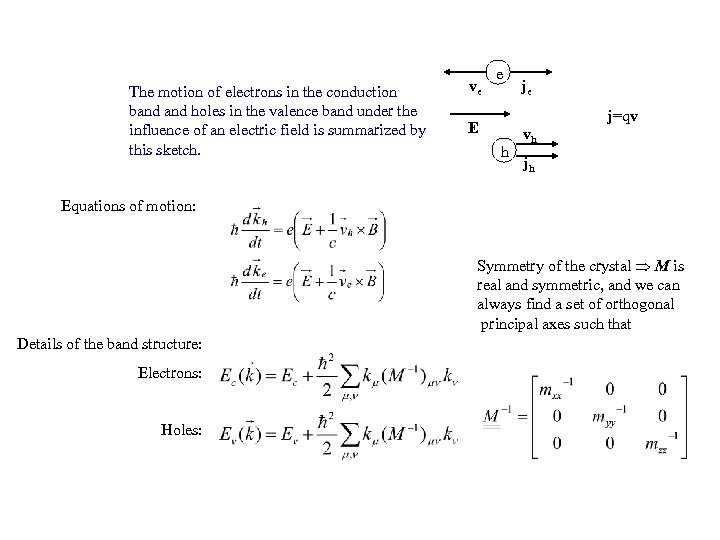The motion of electrons in the conduction band holes in the valence band under the influence of an electric field is summarized by this sketch. ve e je j=qv E h vh jh Equations of motion: Symmetry of the crystal M is real and symmetric, and we can always find a set of orthogonal principal axes such that Details of the band structure: Electrons: Holes:Consider band structure of Si, Ge, Ga. As: me For Ga. As, CBM is at k=0 (i. e. direct bandgap) E Consider spin-orbit interactions for the p-like hole bands. We need to consider addition of angular momentum for orbit and spin: s-like Eg J = L + S with L = 1 S = 1/2 k Heavy Holes (hh) mhh mlh Light Holes j = 3/2 and 1/2 For j=3/2, mj = 3/2 and 1/2 p-like mhh mlh msoh Split-off holes For j=1/2 mj = 1/2 (split-off) s-like Curvature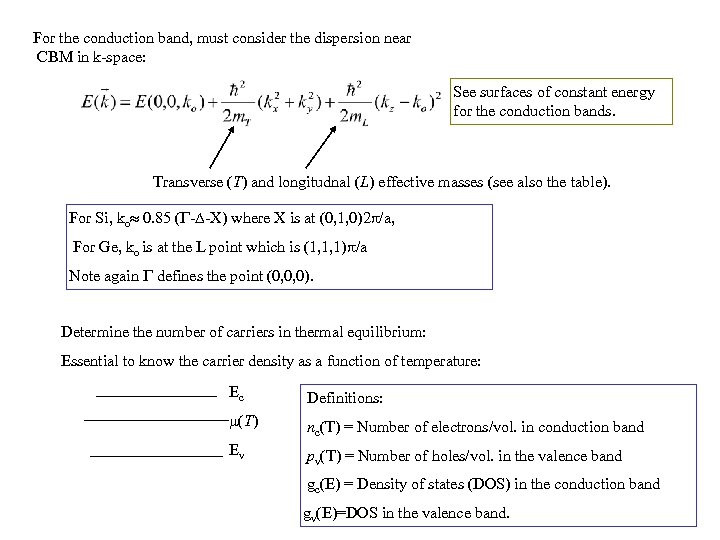For the conduction band, must consider the dispersion near CBM in k-space: See surfaces of constant energy for the conduction bands. Transverse (T) and longitudnal (L) effective masses (see also the table). For Si, ko 0. 85 ( - -X) where X is at (0, 1, 0)2 /a, For Ge, ko is at the L point which is (1, 1, 1) /a Note again defines the point (0, 0, 0). Determine the number of carriers in thermal equilibrium: Essential to know the carrier density as a function of temperature: Ec Definitions: (T ) nc(T) = Number of electrons/vol. in conduction band Ev pv(T) = Number of holes/vol. in the valence band gc(E) = Density of states (DOS) in the conduction band gv(E)=DOS in the valence band.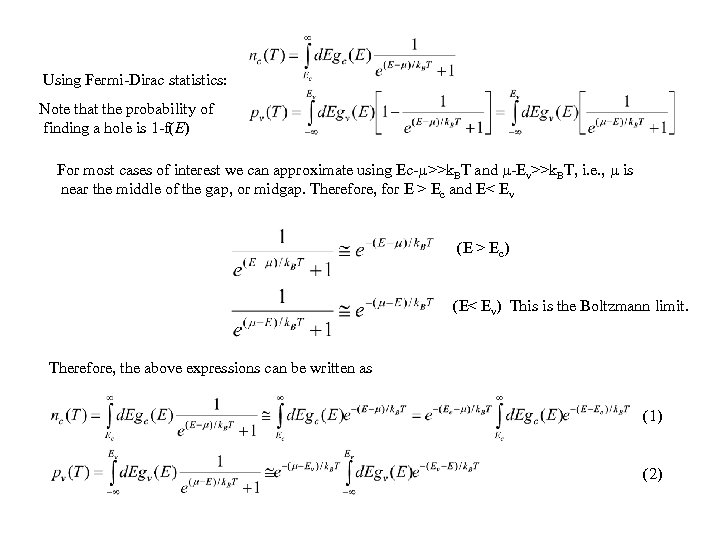Using Fermi-Dirac statistics: Note that the probability of finding a hole is 1 -f(E) For most cases of interest we can approximate using Ec- >>k. BT and -Ev>>k. BT, i. e. , is near the middle of the gap, or midgap. Therefore, for E > Ec and E< Ev (E > Ec) (E< Ev) This is the Boltzmann limit. Therefore, the above expressions can be written as (1) (2)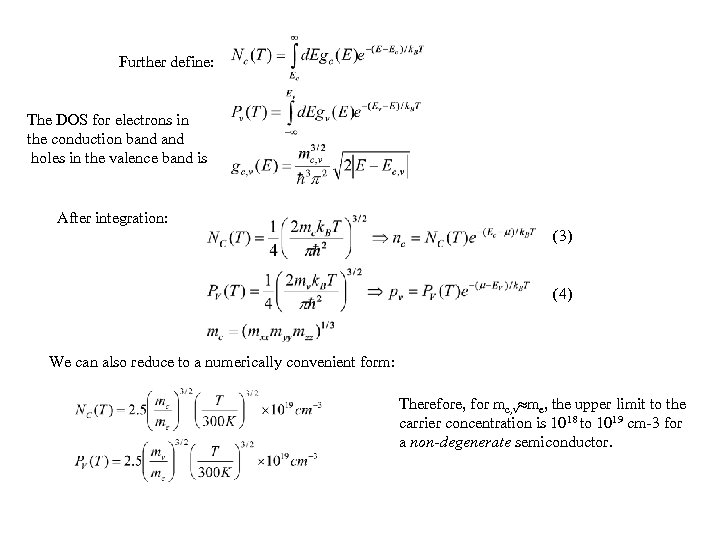Further define: The DOS for electrons in the conduction band holes in the valence band is After integration: (3) (4) We can also reduce to a numerically convenient form: Therefore, for mc, v me, the upper limit to the carrier concentration is 1018 to 1019 cm-3 for a non-degenerate semiconductor.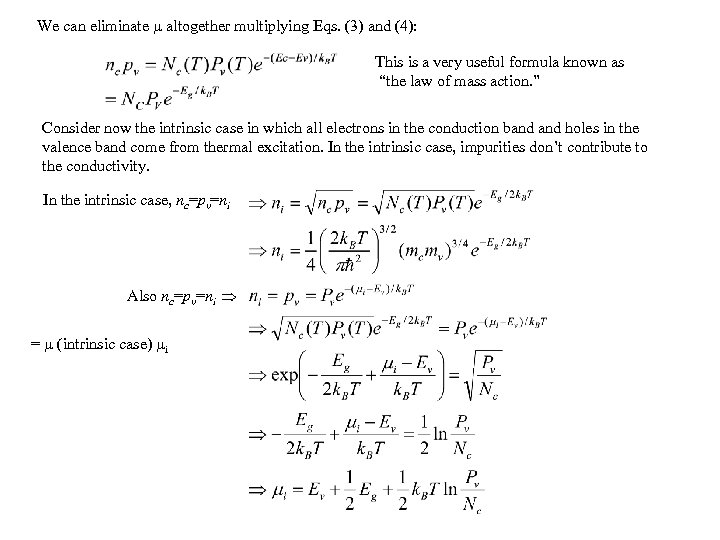We can eliminate altogether multiplying Eqs. (3) and (4): This is a very useful formula known as “the law of mass action. ” Consider now the intrinsic case in which all electrons in the conduction band holes in the valence band come from thermal excitation. In the intrinsic case, impurities don’t contribute to the conductivity. In the intrinsic case, nc=pv=ni Also nc=pv=ni = (intrinsic case) i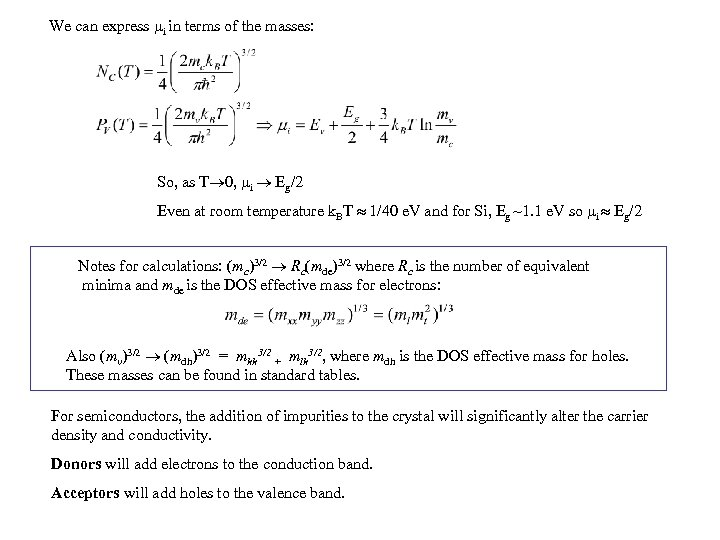We can express i in terms of the masses: So, as T 0, i Eg/2 Even at room temperature k. BT 1/40 e. V and for Si, Eg ~1. 1 e. V so i Eg/2 Notes for calculations: (mc)3/2 Rc(mde)3/2 where Rc is the number of equivalent minima and mde is the DOS effective mass for electrons: Also (mv)3/2 (mdh)3/2 = mhh 3/2 + mlh 3/2, where mdh is the DOS effective mass for holes. These masses can be found in standard tables. For semiconductors, the addition of impurities to the crystal will significantly alter the carrier density and conductivity. Donors will add electrons to the conduction band. Acceptors will add holes to the valence band.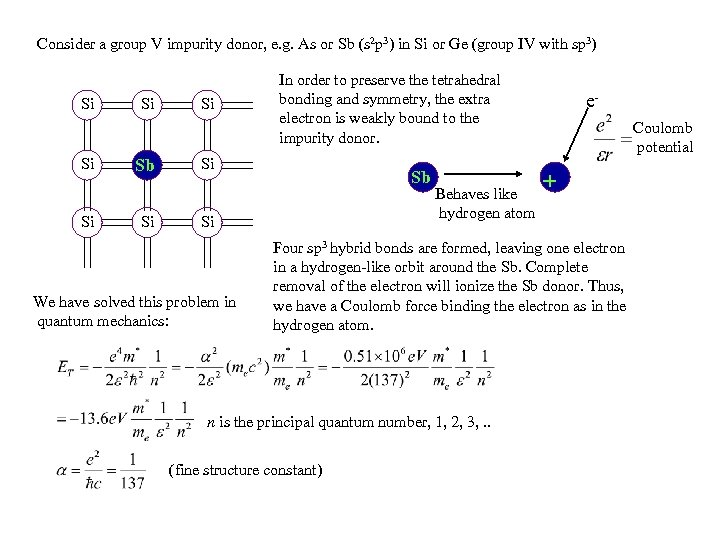Consider a group V impurity donor, e. g. As or Sb (s 2 p 3) in Si or Ge (group IV with sp 3) Si Si Sb Si Si In order to preserve the tetrahedral bonding and symmetry, the extra electron is weakly bound to the impurity donor. Si Sb Si We have solved this problem in quantum mechanics: Behaves like hydrogen atom e. Coulomb potential + Four sp 3 hybrid bonds are formed, leaving one electron in a hydrogen-like orbit around the Sb. Complete removal of the electron will ionize the Sb donor. Thus, we have a Coulomb force binding the electron as in the hydrogen atom. n is the principal quantum number, 1, 2, 3, . . (fine structure constant)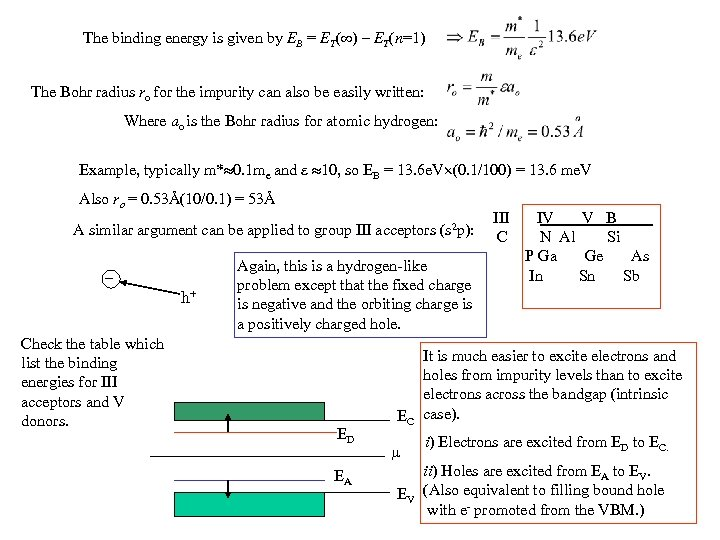The binding energy is given by EB = ET( ) – ET(n=1) The Bohr radius ro for the impurity can also be easily written: Where ao is the Bohr radius for atomic hydrogen: Example, typically m* 0. 1 me and 10, so EB = 13. 6 e. V (0. 1/100) = 13. 6 me. V Also ro = 0. 53Å(10/0. 1) = 53Å A similar argument can be applied to group III acceptors (s 2 p): _ h+ Check the table which list the binding energies for III acceptors and V donors. Again, this is a hydrogen-like problem except that the fixed charge is negative and the orbiting charge is a positively charged hole. ED EA EC EV III C IV V B N Al Si P Ga Ge As In Sn Sb It is much easier to excite electrons and holes from impurity levels than to excite electrons across the bandgap (intrinsic case). i) Electrons are excited from ED to EC. ii) Holes are excited from EA to EV. (Also equivalent to filling bound hole with e- promoted from the VBM. )The statistics for the donors and acceptors are where ND+ is the number of ionized donors, g = 2 (ground state degeneracy of the donor which can have spin up, down, or no electron). where NA- is the number of ionized acceptors, g = 4 since we have spin up/down and two degenerate valence bands which lead to mj = 3/2 and 1/2 (T) will adjust to maintain charge neutrality: Negative charge = Positive charge For an n-type material (i. e. , mostly donors) n = ND+ + p For a p-type material (i. e. , mostly acceptors) p = NA- + n, where n = # of electrons in the conduction band p = # of holes in the valence band. For the partially compensated case: ND+ + p = NA- + n, where both dopants are present.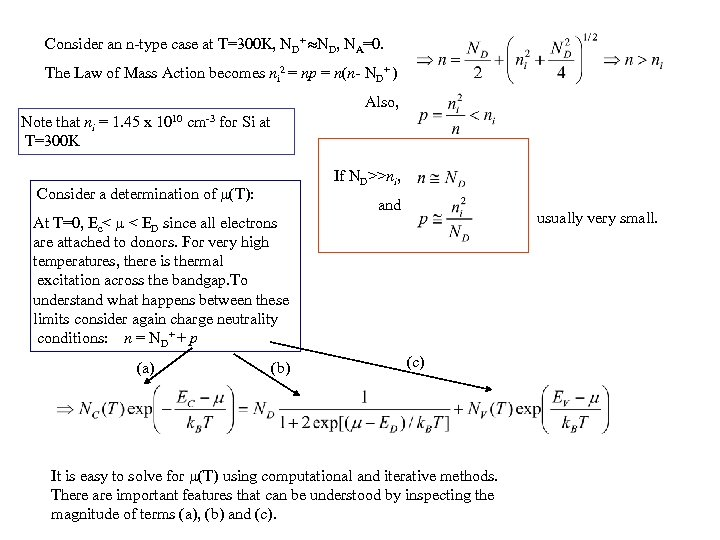Consider an n-type case at T=300 K, ND+ ND, NA=0. The Law of Mass Action becomes ni 2 = np = n(n- ND+ ) Also, Note that ni = 1. 45 x 1010 cm-3 for Si at T=300 K If ND>>ni, Consider a determination of (T): At T=0, Ec< < ED since all electrons are attached to donors. For very high temperatures, there is thermal excitation across the bandgap. To understand what happens between these limits consider again charge neutrality conditions: n = ND+ + p (a) (b) and usually very small. (c) It is easy to solve for (T) using computational and iterative methods. There are important features that can be understood by inspecting the magnitude of terms (a), (b) and (c).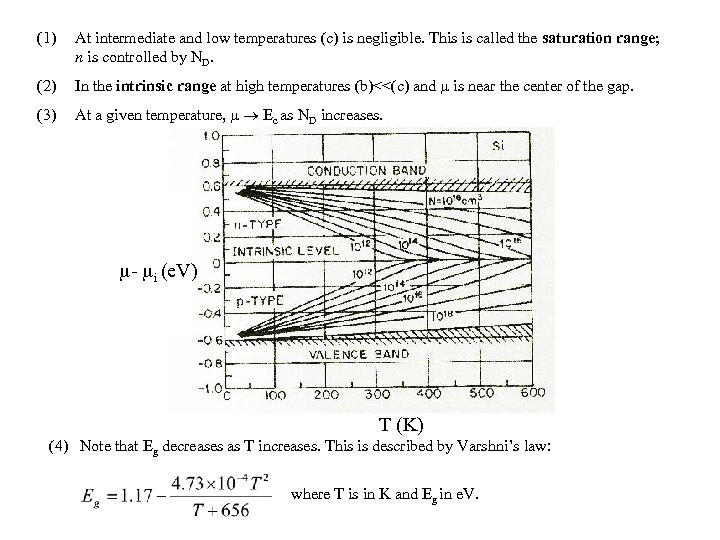(1) At intermediate and low temperatures (c) is negligible. This is called the saturation range; n is controlled by ND. (2) In the intrinsic range at high temperatures (b)<<(c) and is near the center of the gap. (3) At a given temperature, Ec as ND increases. - i (e. V) T (K) (4) Note that Eg decreases as T increases. This is described by Varshni’s law: where T is in K and Eg in e. V.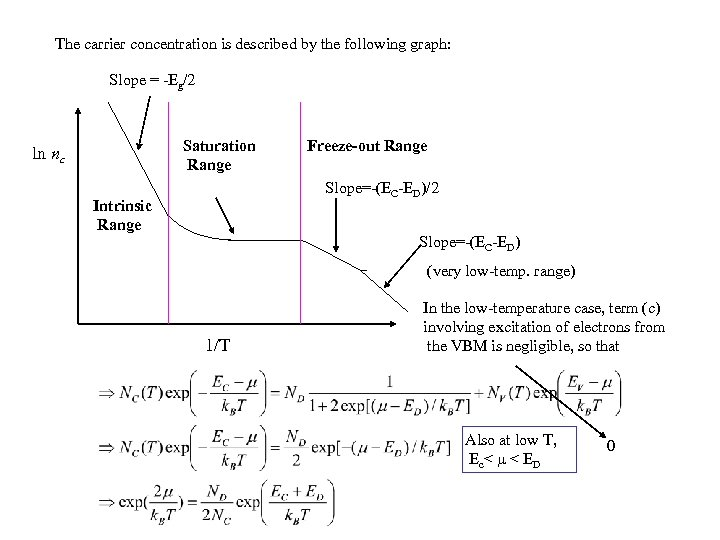The carrier concentration is described by the following graph: Slope = -Eg/2 Saturation Range ln nc Freeze-out Range Slope=-(EC-ED)/2 Intrinsic Range Slope=-(EC-ED) (very low-temp. range) 1/T In the low-temperature case, term (c) involving excitation of electrons from the VBM is negligible, so that Also at low T, Ec< < ED 0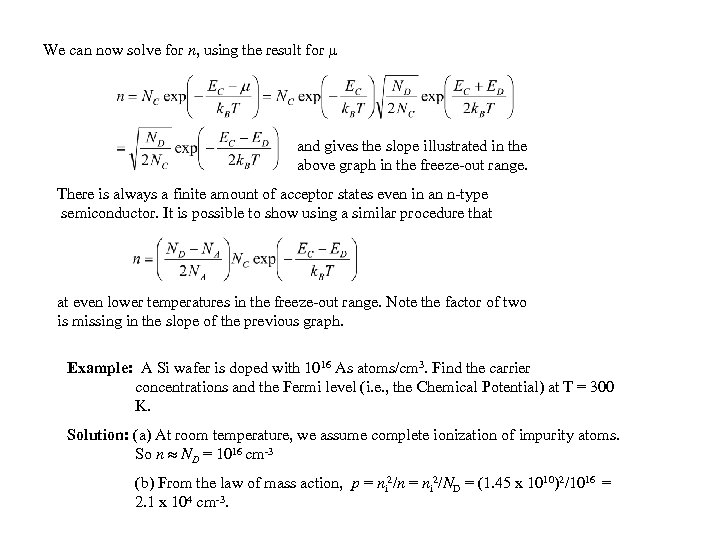We can now solve for n, using the result for and gives the slope illustrated in the above graph in the freeze-out range. There is always a finite amount of acceptor states even in an n-type semiconductor. It is possible to show using a similar procedure that at even lower temperatures in the freeze-out range. Note the factor of two is missing in the slope of the previous graph. Example: A Si wafer is doped with 1016 As atoms/cm 3. Find the carrier concentrations and the Fermi level (i. e. , the Chemical Potential) at T = 300 K. Solution: (a) At room temperature, we assume complete ionization of impurity atoms. So n ND = 1016 cm-3 (b) From the law of mass action, p = ni 2/n = ni 2/ND = (1. 45 x 1010)2/1016 = 2. 1 x 104 cm-3.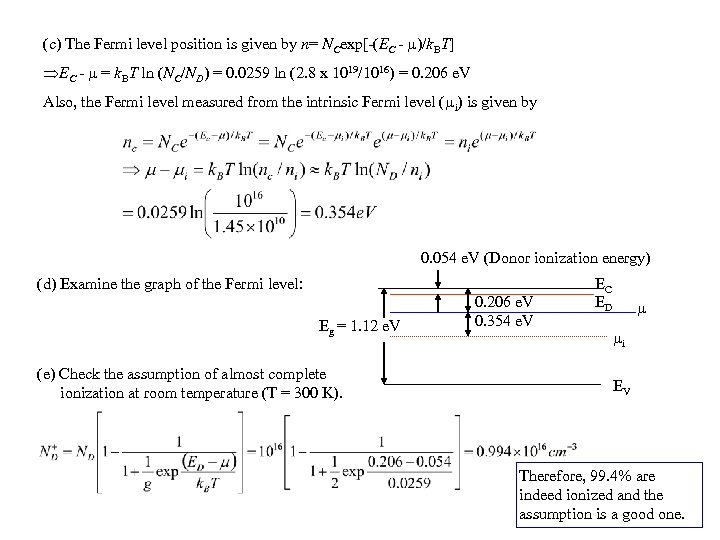(c) The Fermi level position is given by n= NCexp[-(EC - )/k. BT] EC - = k. BT ln (NC/ND) = 0. 0259 ln (2. 8 x 1019/1016) = 0. 206 e. V Also, the Fermi level measured from the intrinsic Fermi level ( i) is given by 0. 054 e. V (Donor ionization energy) (d) Examine the graph of the Fermi level: Eg = 1. 12 e. V (e) Check the assumption of almost complete ionization at room temperature (T = 300 K). 0. 206 e. V 0. 354 e. V EC ED i EV Therefore, 99. 4% are indeed ionized and the assumption is a good one.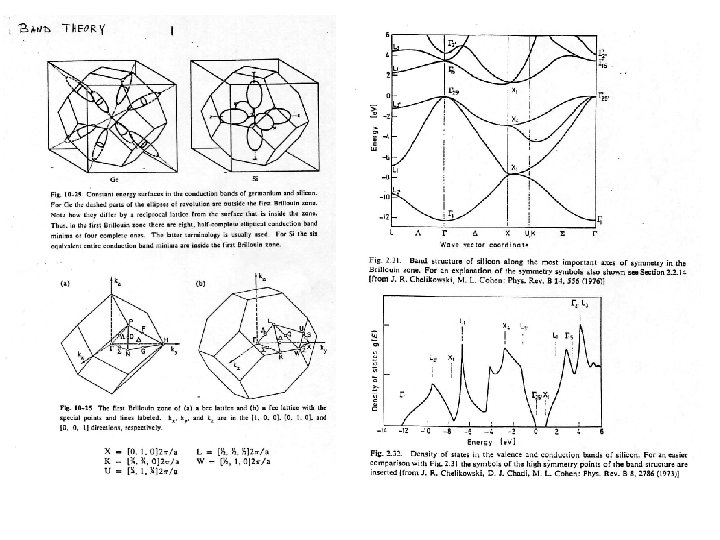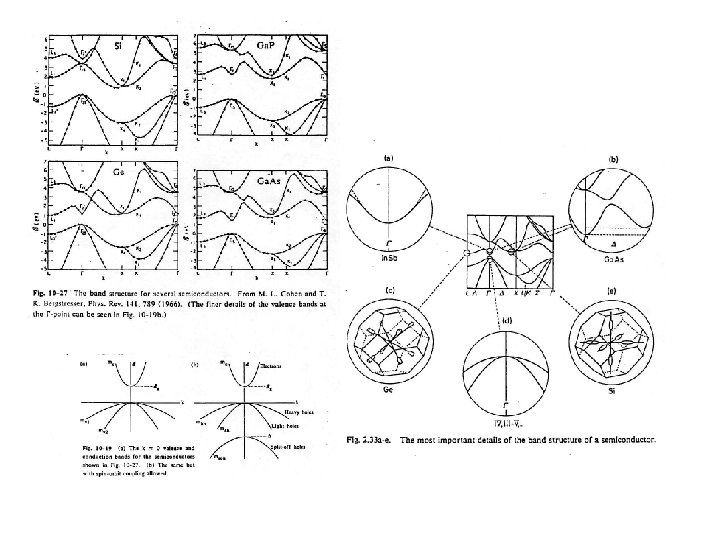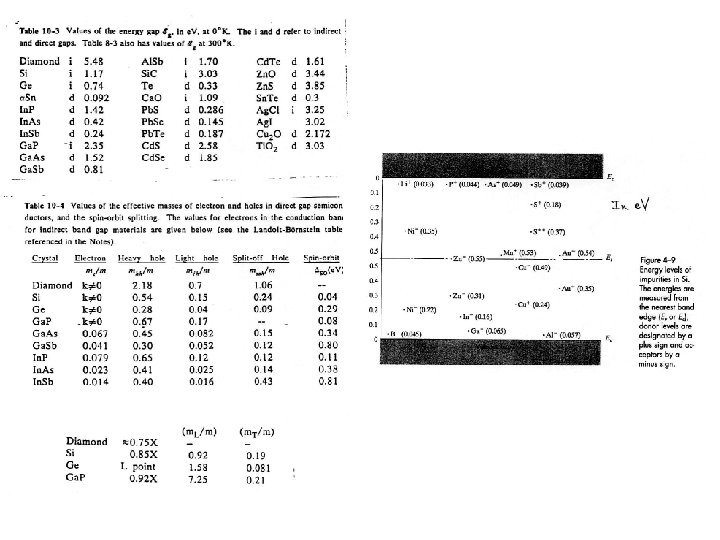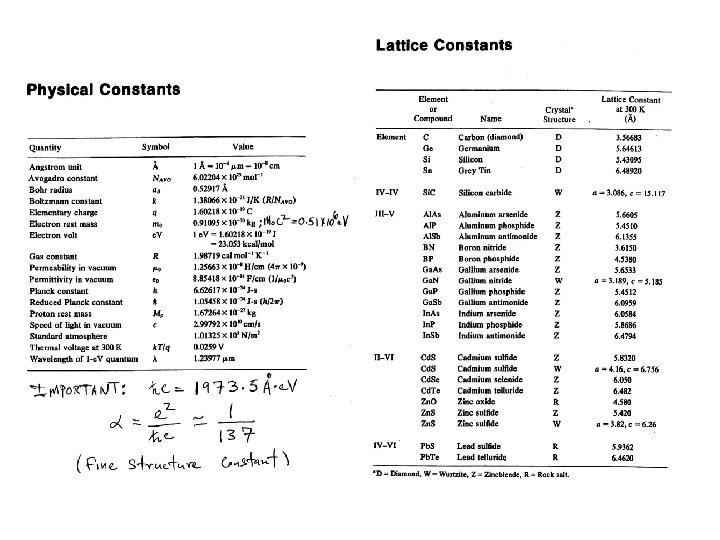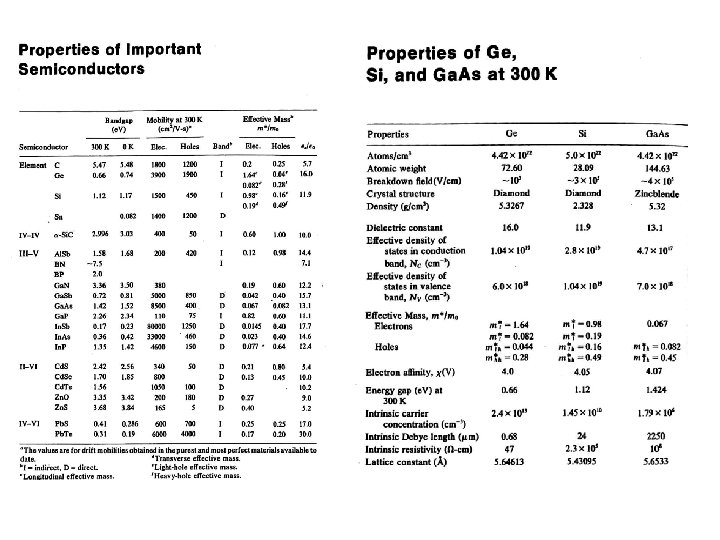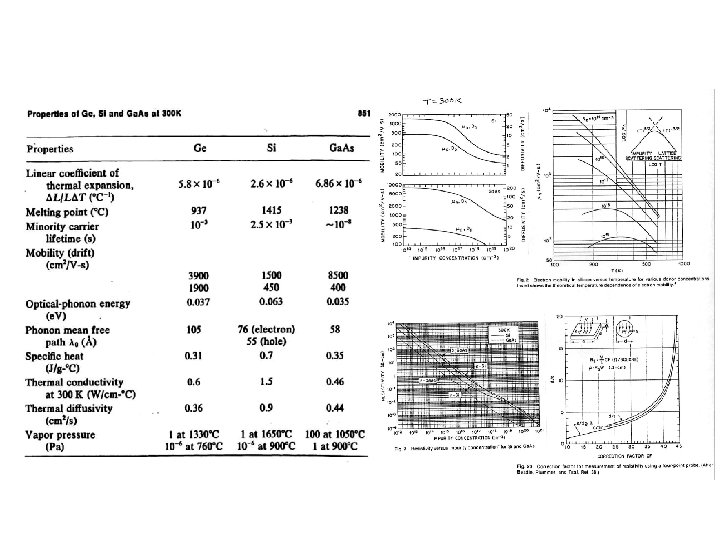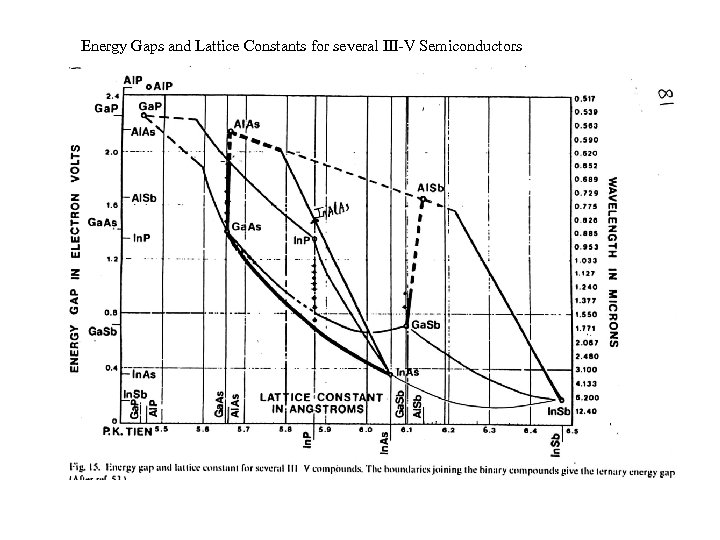Energy Gaps and Lattice Constants for several III-V Semiconductors